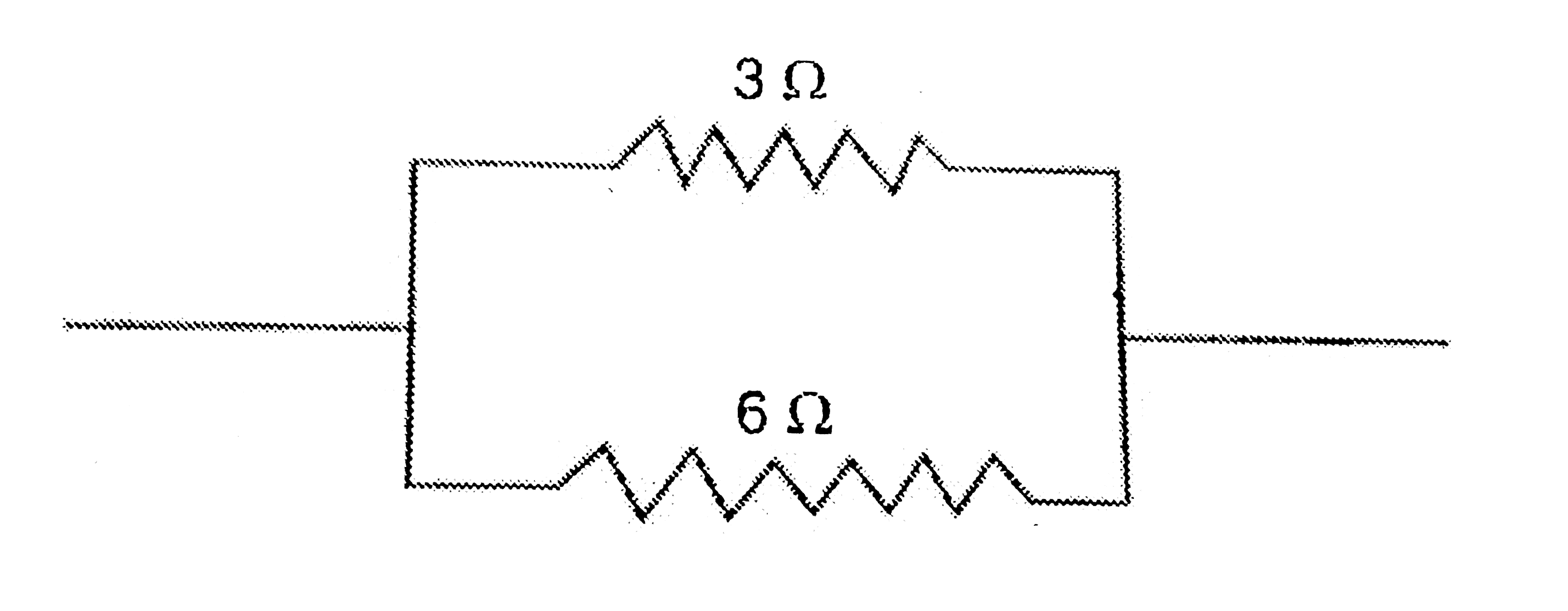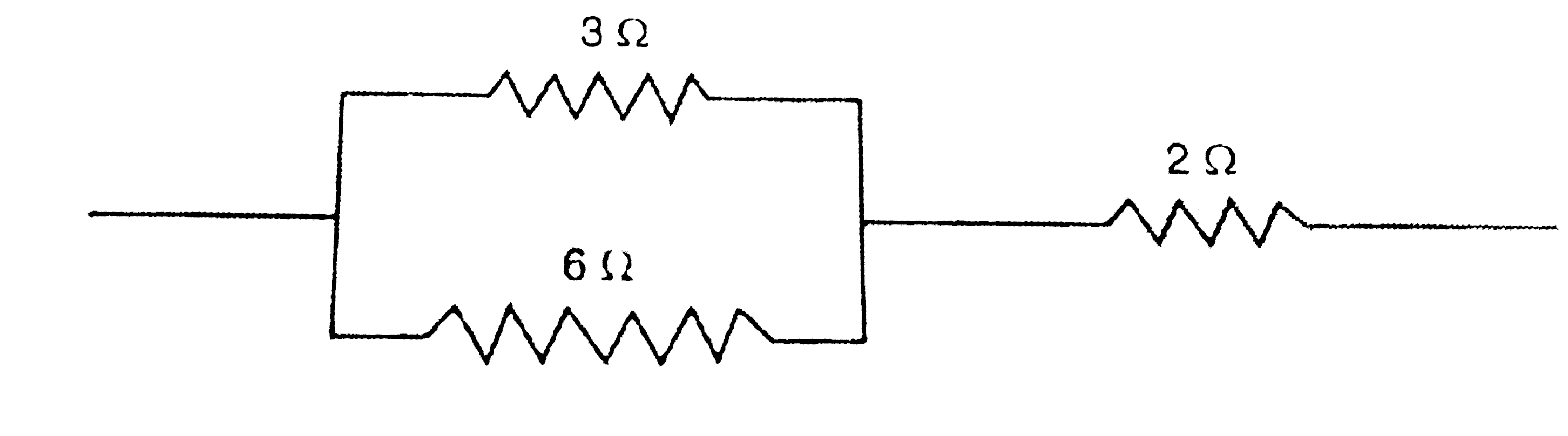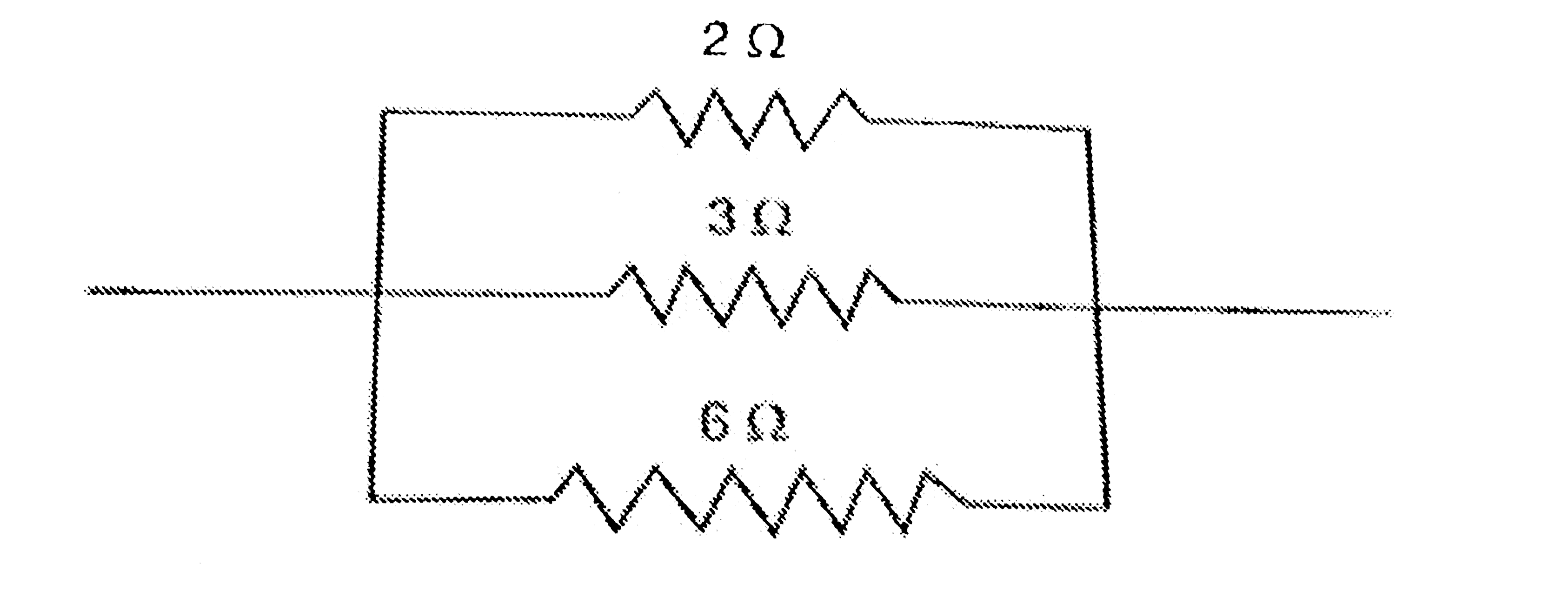# How can three resistors of resistances 2 Omega,3 Omega, and 6 Omega be connected to give a total resistance of (a) 4 Omega (b) 1 Omega ?

73 views
in Physics
closed
How can three resistors of resistances 2 Omega,3 Omega, and 6 Omega be connected to give a total resistance of
(a) 4 Omega
(b) 1 Omega ?

+1 vote
by (67.6k points)
selected by

(a) In order to obtain a total resistance of 4 Omega from three resistors of 2 Omega, 3 Omega and 6 Omega:
(i) First connect the two resistors of 3 Omega and 6 Omega in parallel to get a total resistance of 2 Omega. This is because in parallel combination:
(1)/(R) = (1)/(R_(1)) +(1)/(R_(2))
(1)/(R) = (1)/(3)+(1)/(6)
(1)/(R) =(2+1)/(6)
(1)/(R) = (3)/(6)
R = (6)/(3)
R = 2 Omega(ii) Then the parallel combination of 3 Omega and 6 Omega resistors (which is equivalent to 2 Omega resistance) is connected in series with the remaining 2 Omega resistor to get a total resistance of 4 Omega. This is because in series combination:
R = R_(1)+R_(2)
R = 2 +2
R = 4 Omega
The arrangement of three resistors of 2 Omega, 3 Omega and 6 Omega which gives a total resistance of 4 Omega can now be represented as follows:Thus, we can obtain a total resistance of 4 Omega by connecting a parallel combination of 3 Omega and 6Omega resistors in series with 2 Omega resistor.
(b) In order to obtain a total resistance of 1 Omega from three resistors of 2 Omega, 3 Omega and 6 Omega, all the three resistors should be connected in parallel. This is because in parallel combination:
(1)/(R) = (1)/(R_(1)) +(1)/(R_(2))+(1)/(R_(3))
(1)/(R) = (1)/(2)+(1)/(3)+(1)/(6)
(1)/(R) = (3+2+1)/(6)
(1)/(R) = (6)/(6)
R = (6)/(6)
R = 1 Omega